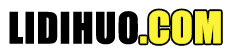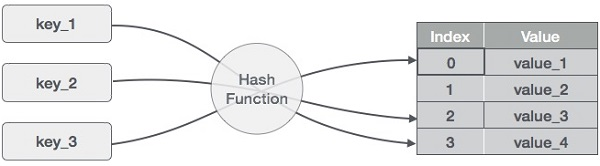# 哈希表

## 散列(1,20) (2,70) (42,80) (4,25) (12,44) (14,32) (17,11) (13,78) (37,98)
 键 哈希 数组索引 1 1 % 20 = 1 1 2 2 % 20 = 2 2 42 42 % 20 = 2 2 4 4 % 20 = 4 4 12 12 % 20 = 12 12 14 14 % 20 = 14 14 17 17 % 20 = 17 17 13 13 % 20 = 13 13 37 37 % 20 = 17 17

## 线性探测

 键 哈希 数组索引 线性探测后，数组索引 1 1 % 20 = 1 1 1 2 2 % 20 = 2 2 2 42 42 % 20 = 2 2 3 4 4 % 20 = 4 4 4 12 12 % 20 = 12 12 12 14 14 % 20 = 14 14 14 17 17 % 20 = 17 17 17 13 13 % 20 = 13 13 13 37 37 % 20 = 17 17 18

## 基本操作

Search-搜索哈希表中的元素。 Insert-在哈希表中插入一个元素。 delete-从哈希表中删除一个元素。

## 数据项

```struct DataItem {
int data;
int key;
};
```

## Hash方法

```int hashCode(int key){
return key % SIZE;
}
```

## Search操作

### 示例

```struct DataItem *search(int key) {
//get the hash
int hashIndex = hashCode(key);

//move in array until an empty
while(hashArray[hashIndex] != null) {

if(hashArray[hashIndex]->key == key)
return hashArray[hashIndex];

//go to next cell
++hashIndex;

//wrap around the table
hashIndex %= SIZE;
}
return NULL;
}
```

## Insert操作

### 示例

```void insert(int key,int data) {
struct DataItem *item = (struct DataItem*) malloc(sizeof(struct DataItem));
item->data = data;
item->key = key;
//get the hash
int hashIndex = hashCode(key);
//move in array until an empty or deleted cell
while(hashArray[hashIndex] != null && hashArray[hashIndex]->key !=-1) {
//go to next cell
++hashIndex;

//wrap around the table
hashIndex %= SIZE;
}

hashArray[hashIndex] = item;
}
```

## 删除操作

### 示例

```struct DataItem* delete(struct DataItem* item) {
int key = item->key;
//get the hash
int hashIndex = hashCode(key);
//move in array until an empty
while(hashArray[hashIndex] !=NULL) {

if(hashArray[hashIndex]->key == key) {
struct DataItem* temp = hashArray[hashIndex];

//assign a dummy item at deleted position
hashArray[hashIndex] = dummyItem;
return temp;
}

//go to next cell
++hashIndex;

//wrap around the table
hashIndex %= SIZE;
}

return NULL;
}
```